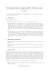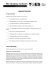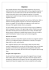# Magnetic Moment - UCSD Department of Physics

## Transcription

Magnetic Moment - UCSD Department of Physics
```CLASSICAL CONCEPT REVIEW 27
Magnetic Moment
If a system of charged particles is rotating, it has a magnetic moment proportional to
its angular momentum, a result from classical electrodynamics sometimes known as
the Larmor theorem. Consider a particle of mass M and charge q moving in a circle of
radius r with speed v and frequency f = v>2pr; this constitutes a current loop. The
angular momentum of the particle is L  Mvr. The magnetic moment of the current
loop is the product of the current and the area of the loop. For a circulating charge, the
current is the charge times the frequency,
i = qf =
and the magnetic moment m is8
m = iA = qa
qv
2pr
v
1
L
b 1pr 2 2 = qa b 2pr
2 M
MM-1
MM-2
From Figure MM-1 we see that, if q is positive, the magnetic moment is in the same
direction as the angular momentum. If q is negative, and L point in opposite directions, that is, they are antiparallel. This enables us to write Equation MM-2 as a vector
equation:
L
q
L
MM-3
2M
Equation MM-3, which we have derived for a single particle moving in a circle, also
holds for a system of particles in any type of motion if the charge-to-mass ratio q>M
is the same for each particle in the system.
The behavior of a system with a magnetic moment in an external (to the system) magnetic field B can be visualized by considering a small bar magnet (Figure
MM-2). When placed in an external magnetic field B, the bar magnet’s magnetic
moment experiences a torque = * B that tends to align the magnet with the
field B. If the magnet is spinning about its axis, the effect of the torque is to make the
spin axis precess about the direction of the external field, just as a spinning top or
gyroscope precesses about the direction of the gravitational field.
To change the orientation of the magnet relative to the applied field direction
(whether or not it is spinning), work must be done on it. If it is moved through angle
du, the work required is
r
v
M
µ
i
MM-1 A particle moving
in a circle has angular
momentum L. If the
particle has a positive
charge, the magnetic
moment due to the current
is parallel to L.
=
dW = t du = mB sin u du = d1 -mB cos2 = d1 - ~ B2
The potential energy of the magnetic moment in the magnetic field B can thus
be written
U = - ~ B
MM-4
78
TIPLER_CCR.indd 78
23/11/11 5:46 PM
Classical Concept Review 27
79
If B is in the z direction, the potential energy is
U = -mz B
(a)
MM-5
B
N
θ
µ
τ=µ×B
S
(b)
B
L
N
θ
µ
τ=µ×B
S
MM-2 Bar-magnet model of magnetic moment. (a) In an external magnetic field, the moment
experiences a torque that tends to align it with the field. If the magnet is spinning (b), the torque
causes the system to precess around the external field.
TIPLER_CCR.indd 79
23/11/11 5:46 PM
```

### Key HW5_2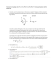### Medizinische Austellung A4 Wien 2008.indd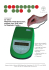### Problem Set 08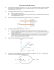### Ph 632 Problem Set #4 - Department of Physics | Oregon State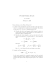### Phys 208 - Recitation E-Fields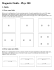### magnasphere - Koloona Industries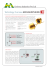### ME-709 Magnetic Recumbent Exercise Bike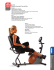### Frontier of Spintronics and Magnetic Sensing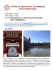### PHYS1250 Fundamental Physics Assignment Three Due Date: 5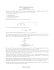### Abstract - MAGNETISM.eu